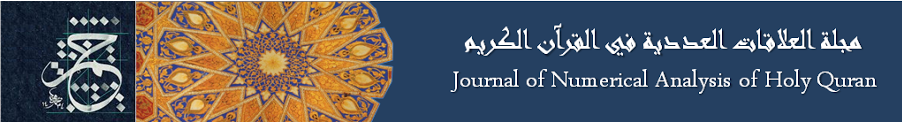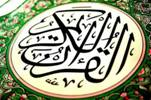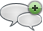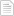### Special numbers of Quran

#### الأربعاء، 22 يوليو 2015 - 0:36The Quran Book has 114 chapters. So N=114 Pivot chapter (Al-7adeed) = N/2 = 57 The pivot chapter is a multiplication of two prime numbers P1*P2=3*19 Number of Pivot chapter verses is prime number P3 = median (1..N/2) = 29 The product of P1*P2*P3 = 3*19*29 = 1653 has the property where the 16th prime = 53أضف تعليقك
##### رجاء ضرورة الإلتزام بعدم إضافة أي تعليق يمس أو يسيء للأديان أو المعتقدات أو المقدسات, كما نرجو ألا يتضمن التعليق السباب أو أي ألفاظ تخدش الحياء والذوق العام

 الأكثر قراءةSilver in Surat AlInsan.ذكر موسى عليه السلام في القرآن.The 17 Suras Ending with the Letter Alef.ولما بلغ اشده.الحديد.نوح عليه السلام.الكهف.Suras Whose Number and Number of Ayas are Both Prime.مزيد من الاثبات ان عاد الثانية هي امريكا.تماثل في القرآن.
الموقع الرسمي حم © 2015, كل الحقوق محفوظة |